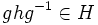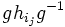Normality is strongly join-closed

This article gives the statement, and possibly proof, of a basic fact in group theory.
View a complete list of basic facts in group theory
VIEW FACTS USING THIS: directly | directly or indirectly, upto two steps | directly or indirectly, upto three steps|
This article gives the statement, and possibly proof, of a subgroup property satisfying a subgroup metaproperty
View all subgroup metaproperty satisfactions | View all subgroup metaproperty dissatisfactions |Get help on looking up metaproperty (dis)satisfactions for subgroup properties
|

Statement

Property-theoretic statement

The subgroup property of being a Normal subgroup (?) satisfies the subgroup metaproperty of being strongly join-closed.

Note: The use of the word strongly is to allow the empty collection as well. We can also say that normality is join-closed and also trivially true.

Verbal statement

The join of an arbitrary (possibly empty) collection of normal subgroups of a group is normal. In other words, the subgroup generated by a family of normal subgroups is normal.

Statement with symbols

Let$I$ be an indexing set and$H_i$ be a family of normal subgroups of$G$ indexed by$I$. Then, the subgroup generated by all the$H_i$, is also a normal subgroup of$G$.

Note that, since any normal subgroup is permutable, the join of a finitely many normal subgroups is equal to their product. Thus, we obtain that if$H, K$ are two normal subgroups of a group$G$, so is the product of subgroups$HK$.

Definitions used

Normal subgroup

Further information: Normal subgroup

A subgroup$N$ of a group$G$ is said to be normal, if given any inner automorphism$\sigma$ of$G$ (i.e., a map sending$x \mapsto gxg^{-1}$ for some$g \in G$), we have$\sigma(N) \le N$. In other words, for any$g \in G, x \in N$, we have$gxg^{-1} \in N$.

Strongly join-closed

A subgroup property is termed strongly join-closed if given any family of subgroups having the property, their join (viz., the subgroup generated by them) also has the property. Note that just saying that a subgroup property is join-closed simply means that given any nonempty family of subgroups with the property, the join also has the property.

Thus, the property of being strongly join-closed is the conjunction of the properties of being join-closed and trivially true, viz., satisfied by the trivial subgroup.

Related facts

• Join lemma for normal subgroup of subgroup with normal subgroup of whole group: If$H$ is a normal subgroup of$G$ and$K$ is a normal subgroup of a subgroup$L$ of$G$, then$\langle H, K \rangle$ is normal in$\langle H, L$. Note that since$H$ is normal, this can be rewritten as:$HK$ is normal in$HL$.
• Normality is strongly intersection-closed: An arbitrary intersection of normal subgroups of a group is a normal subgroup.
• Normality is upper join-closed: If$H \le G$ and$K_i$ is a nonempty collection of subgroups containing$H$ such that$H$ is normal in each$K_i$,$H$ is also normal in the join of the$K_i$s.
• Normality is strongly UL-intersection-closed

Generalizations

The general result (of which this can be viewed as a special case) is that any endo-invariance property is strongly join-closed. This in turn follows from the fact that homomorphisms commute with joins.

Here, an invariance property is the property of being invariant with respect to a certain collection of functions on the whole group. An endo-invariance property is an invariance property with respect to a collection of functions that are all endomorphisms. For normal subgroups, the collection of functions is the inner automorphisms.

Other instances of this generalization are:

Property Endo-invariance property with respect to ... Proof that it is strongly join-closed
Characteristic subgroup automorphisms Characteristicity is strongly join-closed
Fully invariant subgroup endomorphisms Full invariance is strongly join-closed
Strictly characteristic subgroup surjective endomorphisms Strict characteristicity is strongly join-closed
Injective endomorphism-invariant subgroup injective endomorphisms Injective endomorphism-invariance is strongly join-closed

Join-closedness for related subgroup properties

Many subgroup properties closely related to normality fail to be join-closed. For instance:

On the other hand, some properties closely related to normality continue to be join-closed:

Variety of algebras perspective

• Variety of groups is congruence-permutable: The fact that a join of normal subgroups is normal, combined with the fact that the join of normal subgroups equals their product, is closely related to the fact that the variety of groups is congruence-permutable.

Proof

Note that for vacuous reasons, the proofs given here also work when$I$ is empty -- for instance, in the first proof, the product described below has length zero but the arguments hold formally. However, it may be more clear to handle the case of the empty join separately by showing that trivial subgroup is normal.

Proof using conjugation definition

Given: A group$G$ with subgroups$H_i, i \in I$ whose join is a subgroup$H$ of$G$. Further, each$H_i$ is normal in$G$.

To prove: For any$g \in G$ and$h \in H$,$ghg^{-1} \in H$.

Proof: We pick$g \in G$ and$h \in H$.

1. We can write$h = h_{i_1}h_{i_2} \dots h_{i_n}$ where$i_j \in I$ and$h_{i_j} \in H_{i_j}$. This is by the definition of join.
2. We then have that$ghg^{-1} = (gh_{i_1}g^{-1})(gh_{i_2}g^{-1}) \dots (gh_{i_n}g^{-1})$. (This is computationally clear by associativity, but also follows from the conceptual idea that conjugations are automorphisms, i.e., group acts as automorphisms by conjugation).
3. Each$gh_{i_j}g^{-1}$ is in$H_{i_j}$, because$H_{i_j}$ is normal in$G$.
4. From the previous two steps,$ghg^{-1}$ is a product of elements, each of which is in$H_{i_j}$ for some$i_j \in I$ and hence in$H$. Thus,$ghg^{-1} \in H$.

This completes the proof.